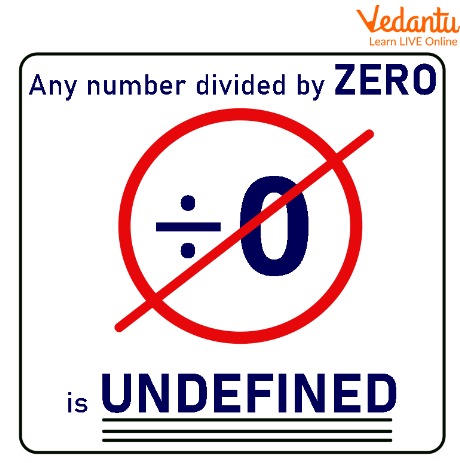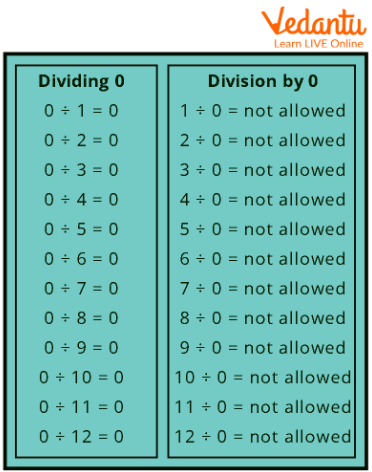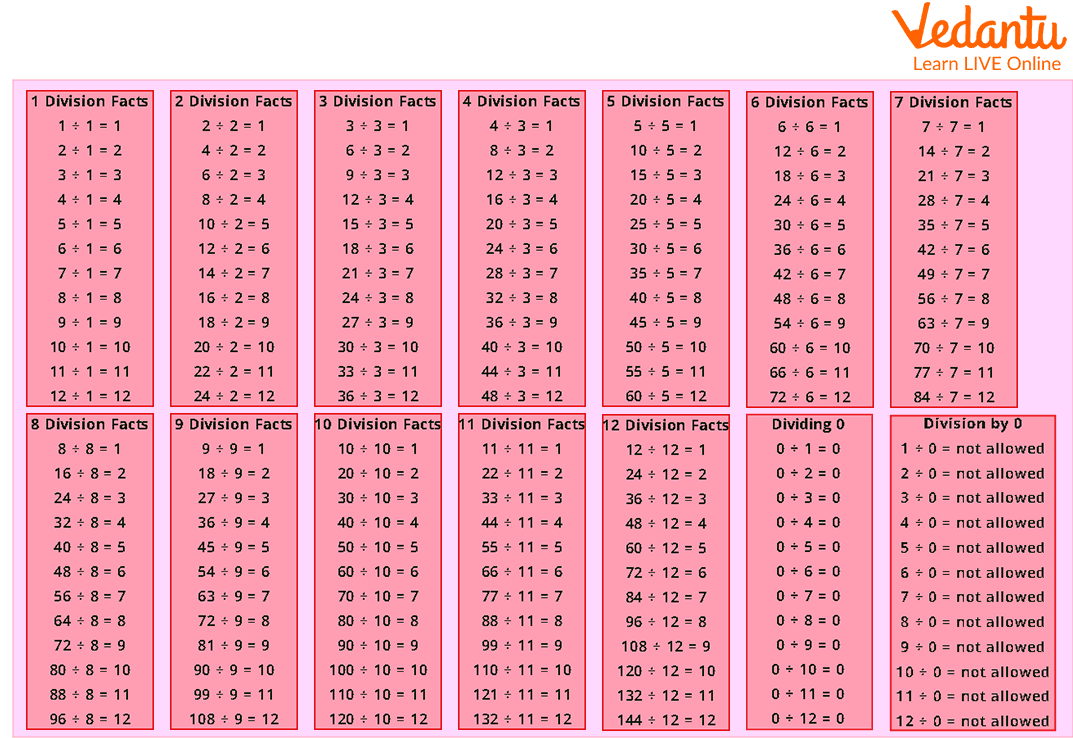Courses
Courses for Kids
Free study material
Free LIVE classes
More

# Division FactsLIVE
Join Vedantu’s FREE Mastercalss

## Introduction

One of the four fundamental arithmetic operations, or how numbers are combined to create new numbers, is division. The other operations are multiplication, addition, and subtraction. At a fundamental level, counting the instances in which one number is included within another is one interpretation of the division of two natural numbers.

Division facts are number phrases that relate to knowing the times tables.

## What is Division Fact?

1. If the dividend is "zero," any number used as a divisor will result in a "zero" quotient.

2. Any dividend will have a quotient equal to itself if the divisor is "1".

3. The dividend is always equal to the product of the divisor and the quotient added to the remainder.

Dividend = (Divisor Quotient) + Remainder.

## Division Facts for Multiplication

A fact involving multiplication is the result of two distinct integers. Additionally, the product is unaffected by the arrangement of the numbers. For instance, $3 \times 2 = 6{\rm { \;and\; }} 2 \times 3 = 6$ .

These days, division facts are frequently taught with multiplication facts as fact families. For instance, a student learning the numbers 2, 3, and 6 would be aware that$3 \times 2 = 6{\rm{ and }}2 \times 3 = 6$, and that $\frac{6}{2} = 3{\rm { and }} \frac{6}{3} = 2$ . No matter how multiplication facts are taught, they can only be learnt via practice. Fortunately, there are a lot of tools available to assist your child master multiplication.

## Division Facts for Zero

Any number used as a divisor will result in a "zero" quotient if the dividend is "zero." A fraction with zero as the denominator is created when a positive or negative number is divided by zero. To express zero divided by a negative or positive value, a fraction with zero as the numerator and the finite amount as the denominator is employed. Divided by it, zero equals zero.Division FactDivision Facts for Zero (Tabular Form)

## Division Facts Examples Image:Division Facts

## Solved Examples(Division Facts with Answers):

Example 1: What is $32 \div 8?$

Ans: We know that $8 \times 4 = 32$

Thus, $32 \div 8 = 4$

Example 2: What is $40 \div 10$ ?

Ans: As we know $10 \times 4 = 40$ ;

Therefore $40 \div 10 = 4$

Example 3: If Shrilly had 49 chocolates and she wants to distribute them in her friends group. There are a total 7 members in the Shrilly group. Help her out in distributing the chocolate so that every member gets an equal number of chocolates.

Ans: Total Number of chocolates Shrilly had = 49

Number of friends = 7

For distributing them $49 \div 7$ chocolates will be the precise way.

As we know, $7 \times 7 = 49$.

Thus, $49 \div 7 = 7$

Therefore, each member will get 7 chocolates.

## Conclusion

One of the four fundamental arithmetic operations is division, which is the process of combining two numbers to create a new number. The remaining operations are multiplication, addition, and subtraction.

Last updated date: 20th Sep 2023
Total views: 81.3k
Views today: 0.81k

## FAQs on Division Facts

1. Could you divide 0?

In mathematics, it is impossible to divide an integer by zero.

2. Each divisor truth is supported by two multiplicative facts.

Two multiplication facts are $2 \times 6 = 12$ and $6 \times 2 = 12$ in the division formula$12 \div 6 = 2$ and $12 \div 2 = 6$.

3. The residue of a division sum is always less than the divisor.

The remainder (2) in the final case is clearly less than the divisor, as can be seen (7).